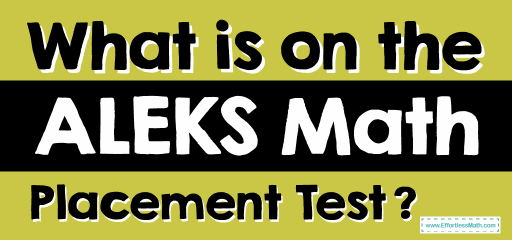# What Is on the ALEKS Math Placement Test?The ALEKS is an artificial-intelligent assessment tool that examines the weaknesses and strengths of a student’s mathematical knowledge, reports its findings to the student, and then provides a learning environment to improve knowledge at the appropriate level for the student’s course.

## The Absolute Best Book to Ace the ALEKS Math Test

The length of the site assessment will vary but can be up to 30 questions. ALEKS Math Assessment ensures that students prepare for specific math courses at the University of Oregon. Because these courses are demanding, students should start the course, which is likely to lead to their success. Students are not allowed to enroll in the math course unless they have demonstrated their readiness.

ALEKS math topics include:

• Real numbers (including integers, fractions, and percentages)
• Equations and inequalities (including linear inequalities, linear equations, systems of linear equations, and quadratic equations)
• Linear and quadratic functions (including linear functions, graphs and functions, and parabolas)
• Exponents and polynomials (including polynomial arithmetic, integer exponents, factoring, and polynomial equations)
• Rational expressions (including rational functions and rational equations)
• Radical expressions (including rational exponents and Root of numbers)
• Exponentials and logarithms (including inverse functions and function compositions, properties of logarithms, and logarithmic equations)
• Geometry and trigonometry (including area, perimeter, and volume, trigonometric functions, coordinate geometry,  and identities and equations)

The ALEKS math provides math formulas for some questions. This test does not provide a list of all the mathematical formulas needed to know the test. So, you should be able to recall many mathematical formulas in ALEKS.

## More from Effortless Math for ALEKS Test …

Check out our FREE ALEKS Math Practice Test.

## Have any questions about the ALEKS Test?

### What people say about "What Is on the ALEKS Math Placement Test? - Effortless Math: We Help Students Learn to LOVE Mathematics"?

No one replied yet.

X
52% OFF

Limited time only!

Save Over 52%

SAVE \$40

It was \$76.99 now it is \$36.99